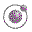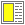Expressions, Types, and Functions - slide 9 : 46

Equality in Scheme
As most other programming languages, Scheme supports a number of different equivalence predicates
• The most discriminating

• eq?

• The least discriminating - structural equivalence

• equal?

• Exact numerical equality

• =

• Others

• eqv? is close to eq?

• string=? is structural equivalence on stringsA sample interaction using and demonstrating the equivalence functions in Scheme.